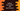# Zip function in python and how to use it### What is zip() :

The syntax of zip() function is ‘zip(*iterables)’_ that means it will take _multiple iterables as inputs. It makes an iterator aggregating elements from each of the _iterable. _

### What it returns :

zip()_ returns an iterator of tuples_. If no argument is passed, it returns an empty iterator._ If only _one argument is passed, it returns an iterator of 1-tuples. i-th tuple contains the _i-th element from each of the _argument sequences or iterables. Iterator stops when _shortest input iterable is exhausted. Following examples will clarify your doubts :_

### Example of python zip() with one argument :

``````#create one list
list = ['one','two','three','four']

#zip the list
zipped_list = zip(list)

#print all the items
for item in zipped_list:
print(item)``````

In this program, we have passed one list to _‘zip()’ and printing out the values of the iterator returned. The output is as below :_

``````('one',)
('two',)
('three',)
('four',)``````

So, 4 tuples are returned.Let’s try with multiple lists

### Example of python zip() with multiple arguments :

``````#create two lists
list_one = ['one','two','three','four']
list_two = ['1','2','3','4']

#zip both lists
zipped_list = zip(list_one,list_two)

#print all the items
for item in zipped_list:
print(item)``````

Output is :

``````('one', '1')
('two', '2')
('three', '3')
('four', '4')``````

Similarly, we can zip three lists :

``````#create three lists
list_one = ['one','two','three','four']
list_two = ['1','2','3','4']
list_three = ['A','B','C','D']

#zip all lists
zipped_list = zip(list_one,list_two,list_three)

#print all the items
for item in zipped_list:
print(item)``````

Output :

``````('one', '1', 'A')
('two', '2', 'B')
('three', '3', 'C')
('four', '4', 'D')``````

### Example of python zip() with different length arguments :

``````#create three lists
list_one = ['one','two','three','four','five','six','seven']
list_two = ['1','2','3','4','5','6']
list_three = ['A','B','C','D']

#zip all lists
zipped_list = zip(list_one,list_two,list_three)

#print all the items
for item in zipped_list:
print(item)``````

In the above example, we have three variables with different length.The output looks like :

``````('one', '1', 'A')
('two', '2', 'B')
('three', '3', 'C')
('four', '4', 'D')``````

Not all items are included from the first and second list !_ This is because the iterator stops when the shortest input iterable is exhausted_ . So the number of tuple is same as the __minimum length of the input argument.

### Example of python zip() with different type of arguments :

In our previous examples, we used only ’list_’ as argument. But we can also pass different type of arguments to the ’zip()’_ function. Example :__

``````#create one list and one tuple
my_list = ['one','two','three','four']
my_tuple = ('1','2','3','4')

#zip both arguments
zipped_list = zip(my_list,my_tuple)

#print all the items
for item in zipped_list:
print(item)``````

It will print the following output :

``````('one', '1')
('two', '2')
('three', '3')
('four', '4')``````

So, output is same as previous.

### Unzipping values :

Let’s try to unzip the values after zipping :

``````#create one list and one tuple
my_list = ['one','two','three','four']
my_tuple = ('1','2','3','4')

#zip both arguments
zipped_list = zip(my_list,my_tuple)

first,second = zip(*zipped_list)

print(first)
print(second)``````

Output :

``````('one', 'two', 'three', 'four')
('1', '2', '3', '4')``````

So, we can unzip the zipped values using the same zip() method and passing the argument containing the zipped values. Only difference is that we need to add _one asterisk(*) before the argument name._